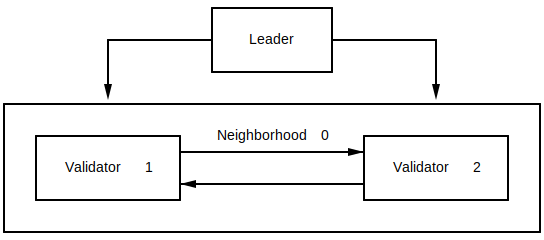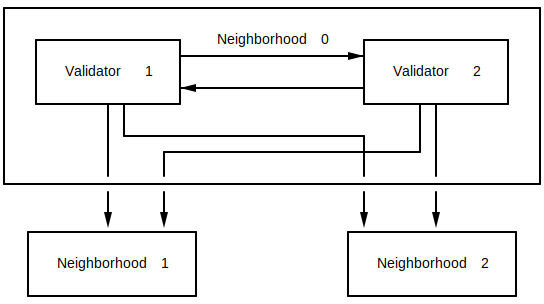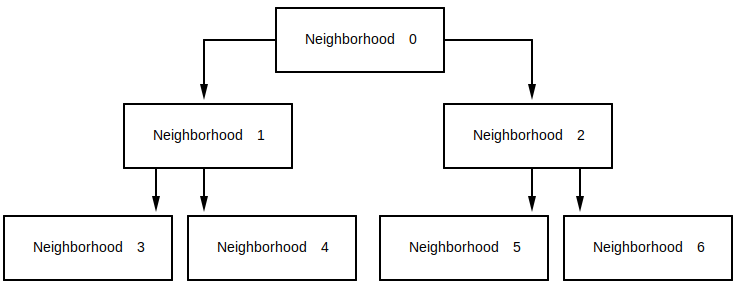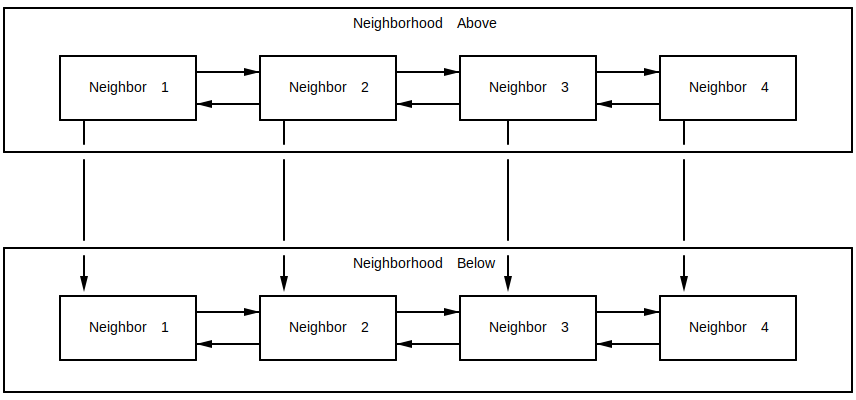# 涡轮（Turbine）区块传播

Solana 集群使用一个叫做 Turbine 的多层区块传播机制来广播所有节点的交易，该过程只产生少量的重复信息。 群集将自身分成小的节点集合，叫做 neighborhoods（邻居）。 每个节点负责与其附近的其他节点分享它收到的任何数据，同时将数据传播到其他邻居的小节点。 这样，每个节点只需要与少数节点通信。

## 层和邻居结构#### 设置值#

DATA_PLANE_FANOUT - 确定层 0 的大小。 后续的层由 DATA_PLANE_FANOUT 系数生成。 邻居的节点数量等于扩散值。 某个邻居要在添加新邻居之前被填满，即如果某个邻居没有满，它必须是最后一个。

## 计算所需的 FEC 比率#

Turbine 依赖于验证器之间数据包的再传输。 由于重新传输，任何网络宽包丢失都会加重， 同时每次访问的数据包未能到达目的地的概率也会增加。 FEC 比率需要考虑网络宽数据包丢失和传播深度。

• 数据包失败的概率为： P = 1 - (1 - network_packet_loss_rate)^2
• FEC 率： K:M
• 尝试次数： N = K + M
• 碎片组失败率: S = SUM of i=0 -> M for binomial(prob_failure = P, trials = N, failures = i)
• 每个区块的碎片: G
• 区块成功率： B = (1 - S) ^(G / N)
• i 的精确结果的双向分布被定义为 (N choose i) * P^i * (1 - P)^(N-i)

• 网络丢包率为 15%。
• 50k Tps 的网络每秒产生 6400个碎片。
• FEC 率增加了每个块按 FEC 笔录的总碎片数。

• G = 8000
• P=1 - 0.85 * 0.85 = 1 - 0.7225 = 0.2775
• S = i 的总和 =0 -> 4 为二项分布(prob_fail= 0.2775, trials = 20, fails = i) = 0.689414
• B = (1 - 0.689) ^(8000 / 20) = 10^-203

• G = 12800
• S = i 的总和 =0 -> 32 为二项分布(prob_fail= 0.2775, trials = 64, fails = i) = 0.002132
• B = (1 - 0.002132) ^(12800 / 32) = 0.42583

• G = 12800
• S = i 的总和 =0 -> 32 为二项分布(prob_fail= 0.2775, trials = 64, fails = i) = 0.000048
• B = (1 - 0.000048) ^(12800 / 64) = 0.99045

## 邻居（Neighborhoods）#